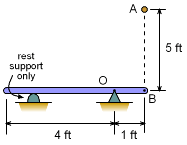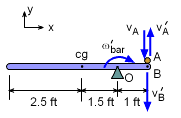Ch 8. Rigid Body Momentum/Impulse Multimedia Engineering Dynamics Impulse Momentum Eccentric Impact
 Chapter - Particle - 1. General Motion 2. Force & Accel. 3. Energy 4. Momentum - Rigid Body - 5. General Motion 6. Force & Accel. 7. Energy 8. Momentum 9. 3-D Motion 10. Vibrations Appendix Basic Math Units Basic Equations Sections Search eBooks Dynamics Fluids Math Mechanics Statics Thermodynamics Author(s): Kurt Gramoll ©Kurt GramollDYNAMICS - EXAMPLERod Impact Example A slender bar, B, has a mass of 2 slugs and is pinned at O. A 2 slug particle, A, is dropped from a height of 5 ft and strikes the bar at the far right end. What is the angular velocity of the bar just after impact? Assume the coefficient of restitution, e, is 1. SolutionVelocity Diagram First, find the velocity of the particle just before it strikes the bar. From conservation of energy,     T1 + V1 = T2 + V2      0.5mvA2 + 0 = 0 + mgh      vA = [32.2(5) / 0.5]0.5 = 17.94 ft/s (down) The impact will transfer the motion of the particle to the motion of the bar attached,      e = [(-v'B) - v'A] / [(-vA) - vB]      1 = (-v'B - v'A) / [-17.94 - 0]      17.94 = v'B + v'A However, the linear velocity of B is related to the angular velocity of the bar,       -v'B = - ω'B rOB       v'B = ω'B (1 ft) Combining gives,       17.94 = ω'B + v'A                             (1) The angular momentum of the bar (with the particle attached) must be conserved, giving,      ΣHo = ΣH'o      mA (-vA) r = mA v'A r + Io (-ω'B)      2 (-17.94)(1) = 2 (v'A)(1) + Io(-ω'B)      -35.89 = 2v'A - Ioω'B                           (2) Mass moment of inertia, Io      Io = mL2/12 + m(1.5)2 = 2(5)2/12 + 2(2.25)      Io = 8.667 slug-ft2                              (3) Combining with Eqs. 1, 2 and 3 gives,      17.94 = ω'B + (-35.89 + 8.667ω'B) / 2 ω'B = 6.729 rad/s

Practice Homework and Test problems now available in the 'Eng Dynamics' mobile app
Includes over 400 problems with complete detailed solutions.
Available now at the Google Play Store and Apple App Store.